# Chemical bonding

In chemistry, a chemical bond is an interaction that keeps the atoms of a molecule together. Pairs of certain atoms can form a stable chemically bonded entity: a diatomic molecule. Examples are two nitrogen atoms chemically bound to the stable dinitrogen molecule (written as N2), two oxygen atoms forming O2, and a hydrogen atom with a fluorine atom forming hydrogen fluoride (HF).

Frequently pairs of bonded atoms form part of a molecule larger than diatomic. For instance, carbon (C) and oxygen (O) form the pair C—O that appears in many organic molecules, from the simplest like methanol H3C—OH to the very complicated deoxyribonucleic acid (DNA). Atoms may also be bound to form a crystal, such as sodium (Na) and chlorine (Cl) that appear in a 1-1 ratio in crystalline rock (kitchen) salt (NaCl). A diamond crystal can be seen as one huge molecule consisting of bonded C—C (carbon-carbon) pairs.

When the total energy of two or more bound atoms is lower than the sum of the energies of the free component atoms, the aggregate of atoms form a stable molecule (or crystal) and the energy lowering is the binding energy of the molecule (or crystal).

It took several centuries before chemical bonding was fully understood, but at present it is generally accepted that non-relativistic quantum mechanical explanations based on Coulomb's electrostatic law give satisfactory accounts of all kinds of bonds. Gravitational forces, strong nuclear forces, or even magnetic forces, do not play any significant role in chemical bonding.

## Types of chemical bonds

Traditionally one distinguishes the following types of bonds:

• Covalent bonds (also known as homopolar bonds). A single covalent bond between two atoms is due to an electron pair shared by the atoms. These bonds are very common in organic chemistry. They occur between, for instance, hydrogen, carbon, nitrogen, and oxygen atoms and give rise to stable, recognizable, molecules. Most covalently bonded molecules remain intact in the solid, liquid, and gaseous aggregation states. Covalent bonding requires quantum mechanics for an explanation and deeper understanding.
• Ionic bonds. Here one atom, say A, loses an electron to its bonding partner, call it B. Hence A becomes the cation A+ and B the anion B. Consecutively, the pair A+B binds strongly through Coulomb interaction. Systems of which the atoms are bound by ionic interactions are usually crystals, the example of rock salt (Na+—Cl) was already mentioned. It requires advanced laboratory techniques to separate ionically bound molecules from crystals, as the crystals are usually very stable. Many ionically bound compounds dissolve in water, dissociating into cations and anions.
• Metallic bonds. A number of metal atoms can crystallize to form a metal, which is a solid recognized by high electric and thermal conductivity. The bonding is caused by delocalized electrons forming electronic bands. The mechanism is akin to the formation of molecular orbitals in molecules. An explanation of metallic bonding is offered by quantum mechanics. Metal molecules (M2, M3, etc.) are not easily prepared experimentally because solid metals are usually very stable.
• Intermolecular (also known as Van der Waals) bonds. These are bonds between stable molecules, see the articles intermolecular forces and Van der Waals forces for more details. Bonds between stable molecules are usually a few orders of magnitude (factors 100 to 10 000) weaker than covalent and ionic bonds. For many years it was believed that hydrogen bonding was to be classified as a separate type of bond, but modern theoretical chemistry recognizes it as a special type of a (fairly strong) intermolecular bond.

## Octet rule

The octet rule is a simple rule that describes the valency of light atoms (atomic number Z ≤ 18, first and second row of the periodic system). The valency of an atom is the number of covalent bonds that the atom can make with a partner. The octet rule starts with the assumption that valency involves only the electrons in the outer shells of the (lowest-energy-state) atoms participating in the bonding. The following lists the number of valence electrons of the first 18 chemical elements

 H(1) He(2) Li(1) Be(2) B(3) C(4) N(5) O(6) F(7) Ne(8) Na(1) Mg(2) Al(3) Si(4) P(5) S(6) Cl(7) Ar(8)

The assumption is that the electron configurations of noble gases (He, Ne, Ar) are particularly stable (since these gases are chemically inert) and that a bonded atom strives after the configuration of a noble gas near to it in the periodic table. Except for hydrogen, lithium, beryllium, and borium, which strive for a helium configuration of two electrons (a duplet), it means that most atoms try to surround themselves with an octet of eight electrons. Of course electrons must be shared with bonding partners to achieve such a noble gas configuration.

In the figure four molecules are shown as examples. Both the Lewis representation (electrons as dots) and the corresponding traditional chemical representation (chemical bonds as lines) are shown. In the first molecule (methane, CH4) the hydrogen atoms have a helium electronic configuration (are surrounded by two electrons) and the carbon has a neon configuration (is surrounded by an octet consisting of eight electrons). In the computation of the formal charge on the atoms it is assumed that the electrons in a bond are shared equally by the bonding partners. Thus, in methane the hydrogen atoms have one electron, while the carbon atom has four. From the above table it follows that the hydrogens and the carbon are formally electrically neutral in CH4. The ethene (formerly known as ethylene) molecule shows a double bond between the carbon atoms. Note that both carbons have a neon configuration (are surrounded by four electron pairs). The next molecule (the nitrogen molecule N2) shows a triple bond. Both N-atoms are surrounded by an octet (four electron pairs). Each N-atom has five electrons and is formally neutral. The last molecule (trimethyloxamine) has as central atom a nitrogen atom with four electrons, and hence the nitrogen lacks an electron and has a formal charge +1. The oxygen atom has three pairs to itself and shares one pair, carrying seven electrons, so that oxygen has the formal charge −1. The total trimethyloxamine molecule is neutral.

### Historical note

The octet rule is usually attributed to Gilbert Newton Lewis, who referred to it as the "rule of eight". The term "octet rule" is due to Irving Langmuir,  who extended and refined the work of Lewis. Lewis saw an atom as a cube with—depending on the atom—one to eight valence electrons on its corners. In this picture molecules consist of cubes piled on top of each other, or next to each other sharing a side or a corner. In Ref. it is argued that this idea is not as novel as it is usually believed, but that the idea of the rule of eight was floating among contemporary chemists and that, for instance, the German chemist Richard Abegg presented already in 1902 some bonding concepts that came close to Lewis's 1916 ideas. In any case, it is generally accepted that Lewis was the first to see the importance of electron pairing. Lewis made the simple assumption that molecules are held together by the pairs of electrons that are shared between bonding atoms and furthermore he assumed that all atomic "kernels" (nuclei plus inner shell electrons) repel one another; at the bond distance the two opposing forces are in equilibrium.

It is remarkable how far physics and chemistry still were separated during the first two decades of the twentieth century. Neither Lewis nor Langmuir put much faith in the Rutherford-Bohr planetary model of the atom in which the negative electrons orbit a positive nucleus. Both chemists believed electrons to be stationary on the vertices of cubic atoms. On the other hand, the founding fathers of quantum mechanical bonding theory (the physicists Walter Heitler, Fritz London, Georg Rumer, Edward Teller, and others) completely ignored the chemists (or perhaps were not even aware of their work). Linus Pauling deserves the credit for having bridged the two branches of science, which he did in a series of papers in the early 1930s and in his famous 1939 book The Nature of the Chemical Bond that is dedicated to G. N. Lewis. In modern chemistry textbooks, chemical bonding theory is usually presented as a continuous development from Lewis's concepts to the Heitler-London theory for the binding in H2. Such presentation of the development of ideas is a historic simplification.

## Ionic bonds

Two of the determining factors in the forming of an ionic bond between atoms are the ionization energies of the atom becoming a cation and the electron affinities of the atom that receives one or more electrons and becomes an anion.

The ionization energy is the minimum energy needed to remove an electron from an atom. Ionization energies vary throughout the periodic table; the elements with the lowest ionization energies (from which an electron is most easily removed) are found at the lower left of the periodic table, near cesium and francium, and elements with the highest ionization energies are found at the upper right of the table, close to fluorine and helium.

The noble gases lie on the right of the periodic table, and the members of the family that are closest to helium (namely, neon and argon) are among the elements with highest ionization energies, so that their electrons are not readily available for bond formation. For the heavier noble gases, krypton and xenon, the ionization energies become comparable to those of other elements and indeed these elements exhibit some compound formation.

Elements on the left in the periodic table, which have one, two, or three electrons in their valence shell can lose these valence electrons, but ionization of electrons from inner shells requires so much energy that it will never occur in chemical bonding. Hence, in chemical bonds one may encounter Na+, Mg2+, and Al3+, but not, for instance, Mg3+, because this would require removal of an electron from the inner shell of magnesium. In general it costs more energy to remove an electron from a cation than from a neutral atom, which means that the first ionization is smaller (in absolute value) than the second, which is again smaller than the third ionization energy (required to extract an electron from a doubly charged cation).

Electron affinity is the energy change accompanying the attachment of electrons to a neutral atom. It is positive when energy is released upon electron attachment to an atom. This usually happens when an electron occupies an empty orbital in the valence shell of the atom. However, if the incoming electron has to occupy a higher shell because the shells of the neutral atom are filled, then energy must be supplied to attach the electron to form an anion and electron affinity is negative. This is always the case for noble gas atoms. Electron affinities vary through the periodic table, but their periodicity is more complex than that of ionization energies. Electron affinities are generally largest close to the upper right of the periodic table (i.e., near fluorine).

In Lewis's picture, the formation of an ionic bond stems from the transfer of electrons from one atom to another. When such a transfer occurs, all the electrons of an element with low first, second, etc., ionization potentials (on the left in the periodic table) are transferred to the other atom that has high electron affinities (is on the right in the periodic table). The electron-donating atom (the cation) obtains the electron configuration of the noble gas atom to its left and the electron-accepting atom (the anion) gets the configuration of the noble gas atom to its right. For example, the neutral alumina (Al2O3) has—according to Lewis—the electronic configuration (Al3+)2 (O2−)3, that is, all atoms have the electron configuration of neutral neon.

Evidently, there is more to the process than a straightforward consideration of ionization energies and electron affinities. When ions bind by Coulomb force, energy is released and this energy must be large enough to overcome the algebraic sum of ionization energies (costs energy) and electron affinities (delivers energy). The Coulomb interaction is inversely proportional to the distance between ions. Thus, it may happen that at large separations the system consisting of neutral atoms has the lowest energy, because the the affinities of the free acceptor atom are smaller than the ionization potentials of the free electron-donating atom. However, when the two atoms are brought together, a point may be reached at which the lowest total energy is obtained if an electron transfers from one atom to the other. At this distance, and at shorter distances, the ionic complex is the lower-energy species.

Finally, it was already pointed out above for NaCl that an ionic compound is typically a solid formed from an array of alternating cations and anions. The packing of ions and their electrostatic interactions account for the typical features of ionic compounds, such as their high melting points.

## Covalent bonds

Covalent bonds possess two typical characteristics:

1. Directionality
2. Saturation

Directionality means that a chemical bond A—B has a preferred direction in space. Let us consider the methane molecule CH4 as an example. The molecule in equilibrium has the shape of a tetrahedron with the carbon atom in the center and the hydrogen atoms on the four corners. The C—H bonds have well-defined directions; changing the direction of a bond, as occurs during bending vibration, costs energy. Other examples: H2O is non-linear, while CO2 is linear. In both cases it costs energy to bend the molecule away from its equilibrium shape.

Atoms have well-defined valencies, they bind chemically with a well-defined number of other atoms. For instance, carbon has maximum valency 4, meaning that carbon binds with at most 4 hydrogen atoms. The valencies of carbon in CH4 are saturated: the complexes CHn are unstable for n > 4. A fifth hydrogen atom does not bind chemically to CH4.

As stated above, quantum mechanics is needed to explain covalent bonding. Quantum mechanics being necessary and sufficient is borne out by elaborate computer calculations on many kinds of molecules. In these calculations the Schrödinger equation of the molecule is solved numerically, yielding among other things, the binding energy of the molecule. Comparison with corresponding experimental numbers has proved abundantly that quantum mechanics is capable of predicting correct numbers. Clearly, these comparisons prove the sufficiency of quantum mechanics, it turns out that all major effects are accounted for in the calculations. All the many efforts that have been undertaken in the past to explain chemical binding by classical electromagnetic/mechanical theories have failed, thus making the necessity of quantum mechanics very plausible.

A main drawback of computer calculations is that they do not lead to insightful explanations of bonding, the calculations are too complex to follow in detail. A non-computer explanation of the covalent bond is restricted to the simplest molecule, the dihydrogen molecule H2. The quantum mechanical treatment of the covalent (homopolar) binding in H2 was first given by Heitler and London in 1927, who used (the then new) quantum mechanics.

### Heitler-London theory

See also molecular orbitals for an alternative (MO) treatment of the binding in H2.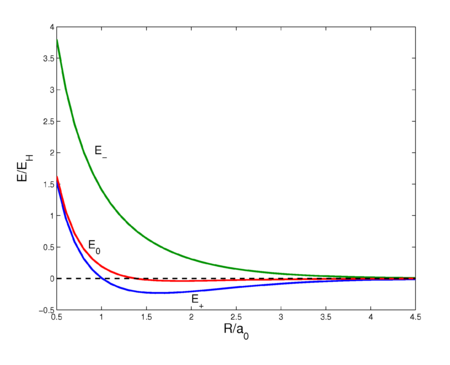Fig. 2. Interaction energy curves as a function of the H—H distance in H2. The Coulomb energy E0 is the energy of the non-symmetrized wave function, E+ is the energy of the symmetric wave function, and E is the energy of the antisymmetric wave function. See this page for the computer code that prepared this plot.

As said, Heitler and London considered the simplest neutral diatomic: the hydrogen molecule. They showed that chemical bonding in this two-electron system is obtained when the typical quantum mechanical notion of the indistinguishability of electrons is accounted for.

Remark: Understanding the remaining part of this section, requires some elementary knowledge of quantum mechanics.

It is evident that if the two hydrogen atoms A and B are isolated from each other by a large separation, the wave function for the two electrons (say electron 1 and electron 2) is simply the product of the two separated wave functions, say ψ(1,2) = φa(1)φb(2) because the Hamiltonian (energy operator) for the two atoms is HA + HB. At close separations, however, ψ(1,2) is only an approximate wave function of the system, because HA + HB is only approximately the Hamiltonian when electron 1 experiences atom B and electron 2 experiences atom A; the non-negligible interaction describing the attraction of electron 1 by nucleus B and electron 2 by nucleus A must be added to the Hamiltonian. Furthermore the two electrons repel each other and this repulsion must also be included when the distance between the atoms is decreasing.

Around the same time (early twentieth century) that it became clear that the motion of electrons has wave character, it was found that electrons are indistinguishable. This implies that the same numbers result from a calculation regardless of which electron is chosen as electron 1 and which as electron 2; Heitler and London refer to this finding as exchange degeneracy.

As a first step Heitler and London used the product of the wave functions of the separate hydrogen atoms as a (approximate) molecular wave function. They computed the expectation value of the energy with respect to this product. The hydrogen atomic wave functions are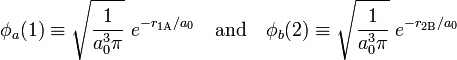$\phi_a(1) \equiv \sqrt{\frac{1}{a_0^3 \pi}}\; e^{-r_{1\mathrm{A}}/a_0} \quad \hbox{and}\quad \phi_b(2) \equiv \sqrt{\frac{1}{a_0^3 \pi}}\; e^{-r_{2\mathrm{B}}/a_0}$

where r1A is the distance of electron 1 to hydrogen nucleus A. Likewise r2B is the distance of electron 2 to nucleus B. The quantity a0 is the Bohr radius (= 52.9 pm = 52.9 10−12 m). The functions are normalized to unity,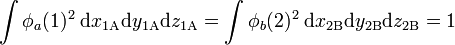$\int \phi_a(1)^2 \; \mathrm{d}x_{1\mathrm{A}}\mathrm{d}y_{1\mathrm{A}}\mathrm{d}z_{1\mathrm{A}} = \int \phi_b(2)^2 \; \mathrm{d}x_{2\mathrm{B}}\mathrm{d}y_{2\mathrm{B}}\mathrm{d}z_{2\mathrm{B}} = 1$

where the integrals are over all of ℝ3. The product function is an eigenfunction of the sum of two hydrogen-atom Hamiltonians, H(0) := HA + HB,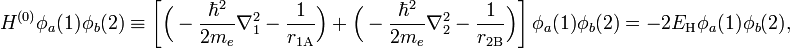$H^{(0)}\phi_a(1)\phi_b(2) \equiv \left[\Big( -\frac{\hbar^2}{2m_e}\nabla_1^2 - \frac{1}{r_{1\mathrm{A}}}\Big) +\Big( -\frac{\hbar^2}{2m_e}\nabla_2^2 - \frac{1}{r_{2\mathrm{B}}}\Big)\right]\phi_a(1)\phi_b(2) = -2E_\mathrm{H} \phi_a(1)\phi_b(2),$

where the electron-nucleus Coulomb interactions are expressed in Gaussian units. Unit of charge is such that electrons have charge −1 and the hydrogen nuclei +1. The quantity ∇2 is the nabla operator squared (acting on the coordinates of electron 1 and 2, respectively); ℏ is Planck's constant over 2π, and me is the mass of an electron. The quantity EH is the energy of one free hydrogen atom in its ground state (≈ 13.6 electronvolt).

The first step of Heitler and London is in fact application of first-order perturbation theory, with H(0) as unperturbed operator and an interaction (perturbation) operator V that consists of Coulomb terms only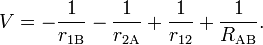$V = -\frac{1}{r_{1\mathrm{B}}}-\frac{1}{r_{2\mathrm{A}}}+\frac{1}{r_{12}} +\frac{1}{R_{\mathrm{AB}}}.$

Here RAB is the distance between the nuclei and r12 is the distance between the electrons. If one interprets φa(1)2 as the charge distribution of electron 1 and φb(2)2 of electron 2, the Coulomb energy between the two (non-polarizable) charge distributions is$E_0(R) \equiv\int\int \phi_a(1)^2 \;V\; \phi_b(2)^2 \; \mathrm{d}x_{1\mathrm{A}}\mathrm{d}y_{1\mathrm{A}}\mathrm{d}z_{1\mathrm{A}}\mathrm{d}x_{2\mathrm{B}}\mathrm{d}y_{2\mathrm{B}}\mathrm{d}z_{2\mathrm{B}}.$

This sixfold integral can be worked out and is a function of RRAB. The result is shown in figure 2 (red curve). The curve shows a very shallow minimum, far less than the known bonding energy (≈ 104 kcal/mol). For shorter distances the curve is repulsive (has negative slope), which is due to the fact that the nucleus-nucleus repulsion is no longer screened by the electrons. Quantum mechanically, E0 is the expectation value of the operator V (first-order energy) with respect to the product wave function φa(1)φb(2). Hence, E0 has two interpretations. Classical: Coulomb interaction between two charge distributions; quantum mechanical: first-order perturbation energy.

A deep quantum mechanical principle was alluded to briefly above: electrons are indistinguishable particles. This may also be phrased as: permutations of electrons are symmetry operators. In this two-electron system there are only two permutations: I  ("doing nothing") and P (interchange of electron 1 and 2). It can be shown that the exact eigenfunctions of a Hamiltonian H (by definition H commutes with its symmetry operators) are adapted to the symmetry of H (technically: eigenfunctions of H belong to irreducible representations of the full symmetry group of H). In the case at hand, it means that the exact spatial eigenfunctions Φ(1,2) of the two-electron system satisfy either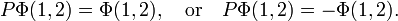$P\Phi(1,2) = \Phi(1,2),\quad\hbox{or}\quad P\Phi(1,2) = -\Phi(1,2).$

The invariant functions are said to be "symmetric" and those obtaining a minus sign are said to be "antisymmetric" under exchange of the coordinates of the two electrons in the wave function.

In order to enforce permutation symmetry on approximate functions, the following short-hand notation is introduced,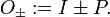$O_{\pm} := I \pm P.$

The projection operators O± have the properties$O_{\pm}^2 = 2O_{\pm}, \quad O_{\pm}^\dagger = O_{\pm},\quad [H,O_{\pm}] = 0.$

(The operators are essentially idempotent, are hermitian operators, and commute with the Hamiltonian.) For conciseness the quantum mechanical bra-ket notation due to P. A. M. Dirac will be used now, for instance,\begin{align} \langle \psi(1,2) | V| \psi(1,2) \rangle &\equiv \langle \phi_a(1)\phi_b(2) | V| \phi_a(1)\phi_b(2) \rangle \\ &= \int\int \phi_a(1)\phi_b(2) \;V\; \phi_a(1)\phi_b(2) \; \mathrm{d}x_{1\mathrm{A}}\mathrm{d}y_{1\mathrm{A}}\mathrm{d}z_{1\mathrm{A}}\mathrm{d}x_{2\mathrm{B}}\mathrm{d}y_{2\mathrm{B}}\mathrm{d}z_{2\mathrm{B}}. \end{align}

Consider the expectation value of total energy operator with respect to the approximate, but symmetrized, wave function. Using the properties of O+, one gets\begin{align} E^{\rm tot}_+ & := \frac{ \langle\; O_+ \psi(1,2) \;|H |\;O_+\psi(1,2)\rangle} { \langle\; O_+ \psi(1,2)\;|\;O_+\psi(1,2)\rangle} = \frac{ 2\langle\; O_+ \psi(1,2) \;|H^{(0)} + V |\;\psi(1,2)\rangle} { 2\langle\; O_+ \psi(1,2)\;|\;\psi(1,2)\rangle} \\ &= 2E_{\rm H} + \frac{ \langle\; O_+ \psi(1,2) \;|V |\psi(1,2)\rangle} { \langle\; O_+ \psi(1,2)\;|\;\psi(1,2)\rangle}. \end{align}

A similar expression holds for the expectation value of H with respect to the antisymmetrized wave function Oψ(1,2). Clearly the function O+ψ(1,2) is symmetric and Oψ(1,2) is antisymmetric under P. These functions have the great advantage over the corresponding non-symmetrized product wave function that they have the same permutation symmetry as the exact wave functions.

Write the interaction energy for the symmetric state as$E_+ = E^{\rm tot}_+ - 2E_{\rm H} = \frac{ \langle\; O_+ \psi(1,2) \;|V |\psi(1,2)\rangle} { \langle\; O_+ \psi(1,2)\;|\;\psi(1,2)\rangle}.$

Similarly for the antisymmetric state,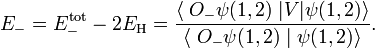$E_- = E^{\rm tot}_- - 2E_{\rm H} = \frac{ \langle\; O_- \psi(1,2) \;|V |\psi(1,2)\rangle} { \langle\; O_- \psi(1,2)\;|\;\psi(1,2)\rangle}.$

Since the atomic functions φa and φb are normalized to unity, it is found that the denominators are,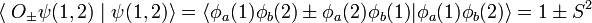$\langle\; O_\pm \psi(1,2)\;|\;\psi(1,2)\rangle = \langle \phi_a(1)\phi_b(2) \pm \phi_a(2)\phi_b(1)| \phi_a(1)\phi_b(2)\rangle = 1 \pm S^2$

where the overlap integral S is given by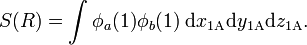$S(R) = \int \phi_a(1)\phi_b(1) \; \mathrm{d}x_{1\mathrm{A}}\mathrm{d}y_{1\mathrm{A}}\mathrm{d}z_{1\mathrm{A}}.$

Hence the Heitler-London interaction energies are$E_\pm = \frac{\langle \phi_a(1)\phi_b(2) \pm \phi_a(2)\phi_b(1) \;|V |\phi_a(1)\phi_b(2)\rangle} { 1\pm S^2}.$

These expectation values, which are a function of internuclear distance R, give reasonable approximations to the exact energies. In figure 2 the following short-hand notation is used,$E_\plusmn(R) = \frac{E_0 \plusmn E_{1} }{1\plusmn S^2}$

with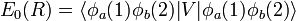$E_0(R) = \langle \phi_a(1)\phi_b(2)|V|\phi_a(1)\phi_b(2)\rangle$

and$E_1(R) = \langle \phi_a(2)\phi_b(1)|V|\phi_a(1)\phi_b(2)\rangle = \int\int \phi_a(2)\phi_b(1) \;V\; \phi_b(2)\phi_a(1) \; \mathrm{d}x_{1\mathrm{A}}\mathrm{d}y_{1\mathrm{A}}\mathrm{d}z_{1\mathrm{A}}\mathrm{d}x_{2\mathrm{B}}\mathrm{d}y_{2\mathrm{B}}\mathrm{d}z_{2\mathrm{B}}.$

The energy E0 was discussed earlier. It is in fact the (classical) Coulomb interaction of two charge distributions, and it is small as long as the internuclear repulsion is screened by the electrons. New is the term E1 which is negative and sizable and the denominator containing the overlap integral S. The presence of E1, often referred to as exchange term, has the consequence that E is strongly repulsive for all R-values, while the energy E+ shows a minimum of 0.232 EH (EH is the energy of one hydrogen atom). The value 0.232 EH ≈ 73 kcal/mol is about 70% of the exact value. The position of the minimum is at 1.64 a0 which is not far from the exact value of 1.49 a0 (a0 is the Bohr radius, a0 = 0.529 10−10 meter).

In conclusion, the exchange term, responsible for the bonding, appears because of the symmetry-adaptation of the zeroth-order wave function. In a classical electrostatic approach, where electrons—as any classical particles—are seen as distinguishable, there is no reason (or possibility) for symmetry-adaptation, the exchange term does not appear, and there is no chemical bonding.

To give an indication of the orders of magnitude the values at 1.60 a0 (near the minimum) are listed:

S = 0.6972, E0 = −0.0307, E1 = −0.3132

At 1.6 a0, the exchange term is an order of magnitude larger than the Coulomb term.

The exchange energy is large mainly due to the nuclear attraction terms in V. One can interpret, for instance, the term$\langle \phi_a(1)\phi_b(2) | -\frac{1}{r_{1B}} | \phi_a(2)\phi_b(1) \rangle = - S\int \frac{\rho_{\rm ex}(1)}{r_{1B}} {\rm d}x_{1B}{\rm d}y_{1B}{\rm d}z_{1B},$

where the "exchange density" of electron 1 is defined by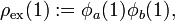$\rho_{\rm ex}(1) := \phi_a(1)\phi_b(1),$

as the attraction of the exchange density by nucleus B. Similarly, the exchange density of electron 2 is attracted by nucleus A. These two attractions are the main cause of the chemical bonding in H2.

#### Remark

The Heitler-London theory is often put forward as a proof of the foresight of G. N. Lewis, who stressed the importance of electron pairs for chemical binding. As pointed out by Slater the hydrogen-molecule-cation H2+, which has one electron, is bound by a mechanism that is very similar to the one responsible for binding in H2. The main difference is that permutation symmetry is replaced by reflection symmetry. Hence one-electron bonds do exist.

#### Relation to electron spin

An electron has a spin up (spin function α) or spin down (spin function β). Two electrons can have four spin functions$\alpha(1)\alpha(2),\quad\alpha(1)\beta(2),\quad \beta(1)\alpha(2),\quad \beta(1)\beta(2)$

These functions are not adapted to permutation symmetry. However, the following linear combinations are adapted\begin{align} \Theta^0_- &= \alpha(1)\beta(2)- \beta(1)\alpha(2) \\ \Theta_+^1 &= \alpha(1)\alpha(2) \\ \Theta_+^0 &= \alpha(1)\beta(2)+ \beta(1)\alpha(2) \\ \Theta_+^{-1} &=\beta(1)\beta(2) \\ \end{align}

It can be shown that these functions are eigenfunctions of the spin angular momentum operators Sz and S2. The one antisymmetric functions is known as a singlet function and the three symmetric functions are known as triplet functions.

Electrons are fermions, which means that their total wave functions (containing spin and space coordinates) must be antisymmetric under permutations. Clearly, the following products of space and spin functions are antisymmetric\begin{align} {} [O_-\phi_a(1)\phi_b(2)]\;\Theta_+^{m} = \psi_-(1,2)\; \Theta_+^{m} &\qquad (m=-1,\;0,\; 1) \\ {} [O_+\phi_a(1)\phi_b(2)]\; \Theta_-^{0} = \psi_+(1,2)\; \Theta_-^{0} & \end{align}

The following products are symmetric (would be valid functions if electrons were bosons, quod non),\begin{align} \psi_+(1,2)\; \Theta_+^{m} &\qquad (m=-1,\;0,\; 1) \\ \psi_-(1,2)\; \Theta_-^{0} \end{align}

Note that ψ+(1,2) (with energy E+) can only occur in conjunction with the spin singlet. The spin singlet state gives binding (sometimes very crudely and bordering to wrong expressed as "opposite spins attract"). The three triplet spin functions combine with ψ(1,2) (with repulsive energies E). Note that Θ0+ also consists of two "opposite spins" and yet combines with the repulsive wave function negating the rule "opposite spins attract".

## Valence bond theory

### General remarks

The Heitler-London approach to the bonding in H2 is the first application of valence bond theory, further developed by Linus Pauling in the 1930s. Valence bond (VB) theory, accounting for chemical bonding in general molecules, has the great advantage that it stays conceptually much closer to chemical bonds—as they are pictured in the minds of chemists—than its competing method, molecular orbital (MO) theory.

However, VB theory is orders of magnitude more difficult to implement on a computer than MO theory. Naively, it would be tempting to assume that valence bond wave functions depend only on the electrons that participate in a certain chemical bond. This is not correct, because all N electrons of a molecule are quantum mechanically indistinguishable and must be handled on equal footing. It is therefore very difficult to translate the Lewis approach—in which certain distinctive pairs of electrons are held responsible for certain bonds—to a consistent quantum mechanical formalism. In principle, valence bond wave functions must be functions of all N molecular electrons and must be adapted to the total permutation group SN consisting of N! permutations. In H2 the number of electrons equals two and since S2 is of order 2, Heitler and London had to consider symmetric and antisymmetric functions only. Clearly, N! rises steeply as function of N and the number of symmetry types (irreducible representations) rises steeply, too.

Not all symmetry types are allowed, though. In the Heitler-London theory it was seen that singlet spin functions combine only with symmetric products of atomic orbitals, whereas triplet spin functions combine only with antisymmetric products of atomic orbitals. This is due to the requirement—first formulated by Wolfgang Pauli—that the total space-spin wave function be antisymmetric. There is a mathematical theory, due to Issai Schur, Alfred Young, Hermann Weyl, and others, that generalizes these ideas and connects spin angular momentum to permutation symmetry. This mathematical theory enables the construction of all-electron valence bond functions (often referred to as valence bond structures) that have the correct permutation and spin symmetry and are antisymmetric. Furthermore, graphical rules exist to choose linearly independent valence bond structure from the very many that can be constructed. As their name suggests, valence bond structures are in one-to-one correspondence with the usual chemical bonding diagrams. Hence they have a clear conceptual advantage over their counterparts in MO theory that are the configurational wave functions constructed as linear combinations of Slater determinants.

A second difficulty in the implementation of valence bond theory on a computer is that valence bond structures, once they have been constructed and a linearly independent set of them has been selected, are composed of antisymmetrized N-electron atomic orbital products. Atomic orbitals on different atoms in a molecule are non-orthogonal. In a typical valence bond calculation it is necessary to evaluate N electron integrals ("matrix elements") of the molecular energy operator H ("Hamiltonian"), which is an N-electron operator. Say Φ and Ψ are valence bond structures, then a VB calculation requires calculation of the following integrals,$\langle \Phi(1,2,\ldots,N) | H | \Psi(1,2,\ldots,N) \rangle = \int\cdots\int \Phi(1,2,\ldots,N)^* H \Psi(1,2,\ldots,N) d\tau_1 d\tau_2\cdots d\tau_N,$

where dτkdxkdykdzk is the volume element corresponding to electron k, k=1, …, N. Due to the antisymmetrization, both Φ and Ψ consist of N! terms, so that the integral consist of (N!)2 terms. In MO theory similar integrals do arise, but there the antisymmetrized products consist of molecular orbitals, which are orthogonal. Due to the orthogonality of the molecular orbitals almost all (N!)2 terms vanish and the N-electron integral is not difficult to compute. However, atomic orbitals being non-orthogonal, in a VB approach practically all (N!)2 terms survive, which makes the integral very time consuming to evaluate. This problem is known as the N-factorial catastrophe.

There is a final problem that valence bond theory shares with molecular orbital theory. In the precomputer era, when integrals were not computed rigorously, it was believed that computations based on a handful of orbital products would suffice. When it became possible to perform computation with the necessary precision it became clear—in VB as well as in MO theory—that extremely many (hundred thousands to millions) antisymmetrized orbital products must be included to obtain results that can be compared with experimental numbers. Moreover, it was found in VB theory that simple atomic orbitals, taken from the free atoms, did not behave as expected. Often atomic orbitals must be prepared in certain ways to function properly in a molecular valence bond calculation.